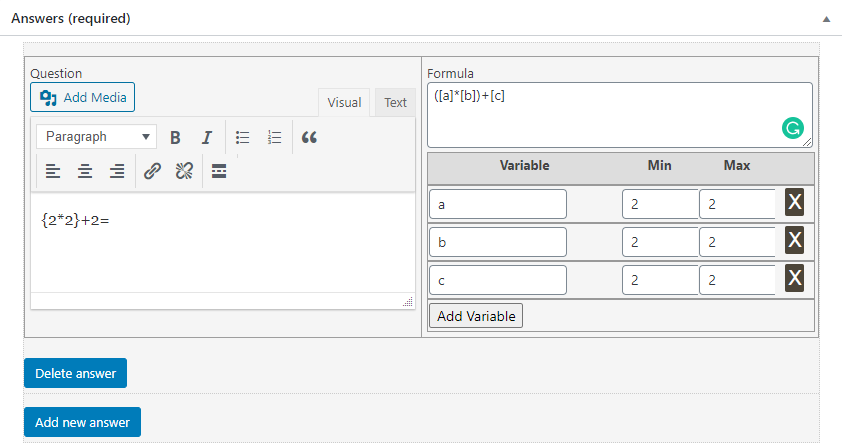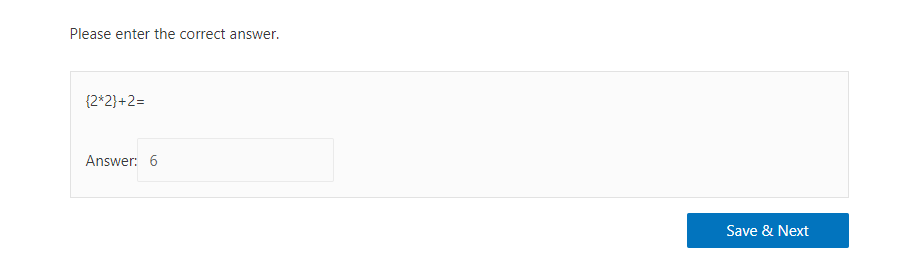LearnDash Arithmetic Quiz - Overview

The LearnDash Arithmetic Quiz add-on adds a new question type to your LearnDash quizzes, allowing you to create more elaborate mathematical evaluations.  The new “Arithmetic” question type” requires  students to solve for a variable by completing a formula.

Installation:

• Install and activate the LearnDash plugin.
• Install and activate the LearnDash Arithmetic Quiz Add-on.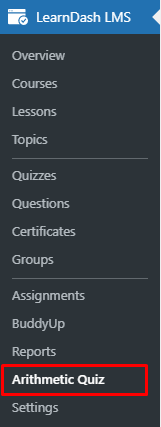Settings: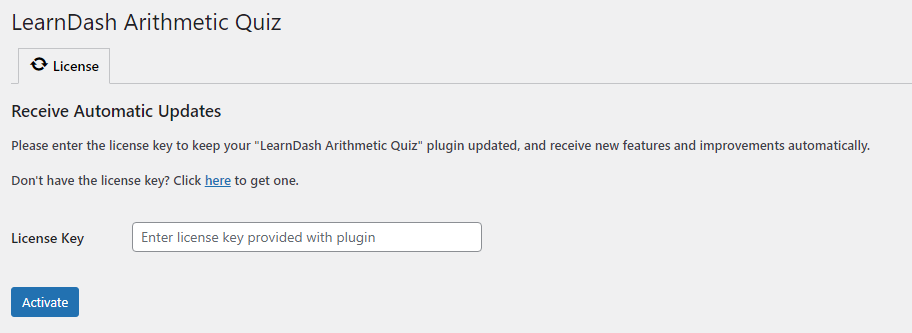• Configuring Arithmetic Questions:

Navigate to LearnDash LMS > Questions and add a new question.

Configure the question’s options following this documentation. Then, select “Arithmetic” from the “Answer Type” meta box on the right and configure the rest of the question’s settings as demonstrated in the following example.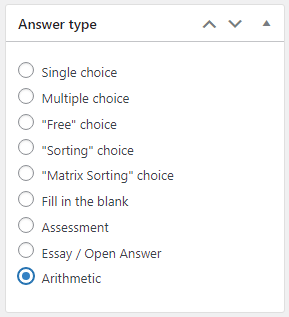Example Question:

“If a small soda glass contains [x] ounces of soda, and a large soda glass contains [y] ounces of soda, what is the total number of ounces in 4 large and 3 small glasses of soda?”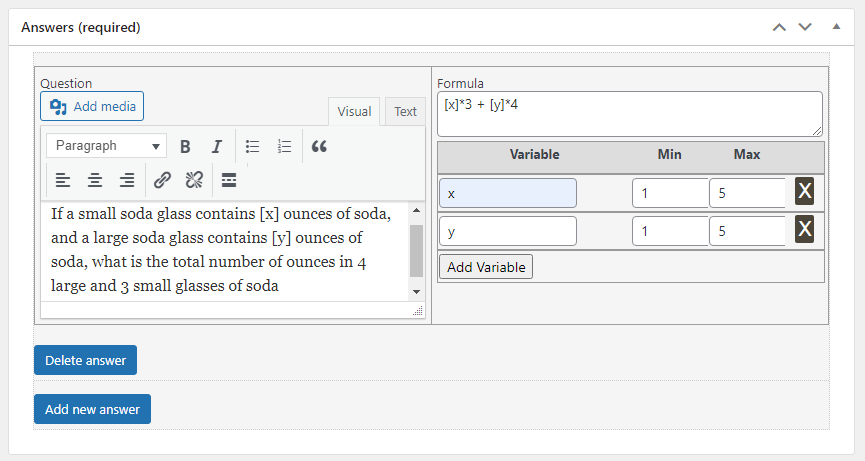Now, if the admin selects a range of 1 - 5 for the variables, the random numbers will be displayed on the frontend against the variable from that range. Enter the same number in the Min and Max field if you want the variables not to be randomized.

This is how this question will be displayed on the frontend: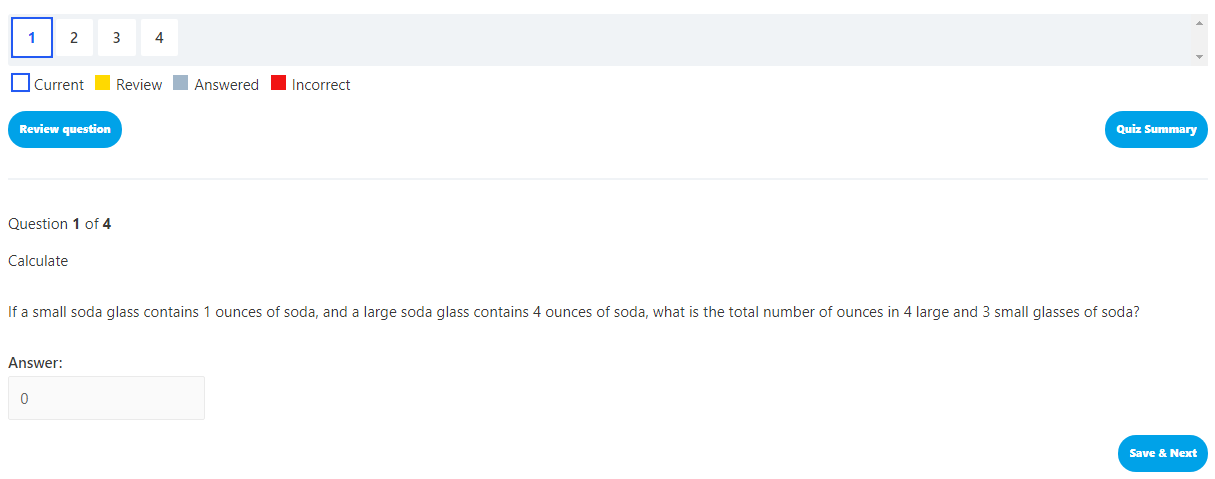Here you’ll find additional examples on how to use this question type to create different kinds of mathematical questions for your LearnDash quizzes:

• Navigate to Questions under the LearnDash menu and click on “Add New”.
• Enter the question title and description.
• Select the answer type as Arithmetic from the Answer Type meta-box.
• Scroll to the Answer(required) section.
• Enter the Question i.e 10 + 2 = _______
• Enter the Formula i.e [x] + [y]
• For this example, enter x as a variableand enter 10 both for Min and Max values. Click on  Add Variable again.
• For this example, enter y as a variable and enter 2 both for Min and Max values.

Note: If you want to randomize the values for a variable, insert the selected value range in min and max fields.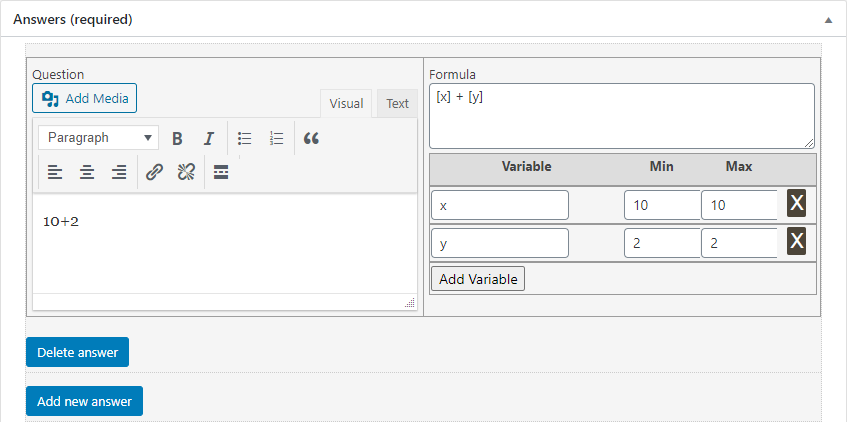This is how the question will be displayed on the frontend: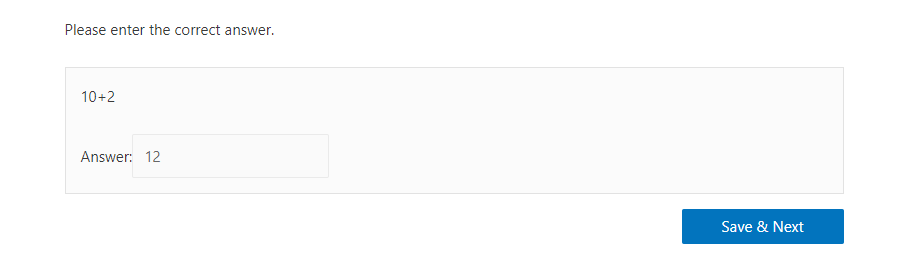Subtraction Questions:

• Navigate to the Questions under the LearnDash menu and click on “Add New”.
• Enter the question title and description.
• Scroll to the Answer(required) section.
• Enter the Question i.e 20 - 10 = _______
• Enter the Formula i.e [x] - [y]
• For this example, enter x as the variable and enter 20 both for Min and Max values.
• For this example, enter y as the variable and enter 10 both for Min and Max values.

Note: If you want to randomize the values for a variable, insert the selected value range in min and max fields.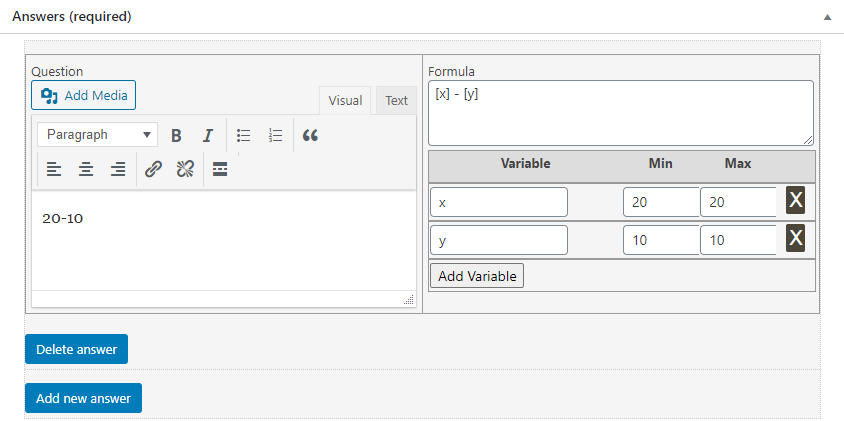This is how the question will be displayed on the frontend: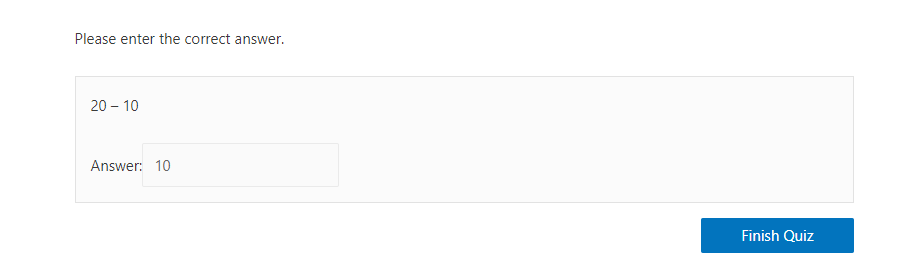Multiplication Questions:

• Navigate to the Questions under the LearnDash menu and click on “Add New”.
• Enter the question title and description.
• Scroll to the Answer(required) section.
• Enter the Question i.e 2 * 2 = _______
• Enter the Formula i.e [x] * [y]
• For this example, enter x as the variable and enter 2 for both the Min and Max values.
• For this example, enter y as the variable and enter 2 for both the Min and Max values.

Note: If you want to randomize the values for a variable, insert the selected value range in min and max fields.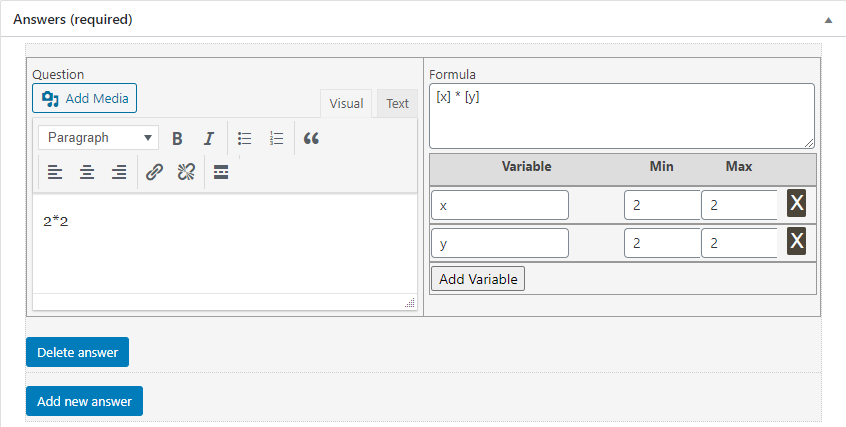This is how the question will be displayed on the frontend: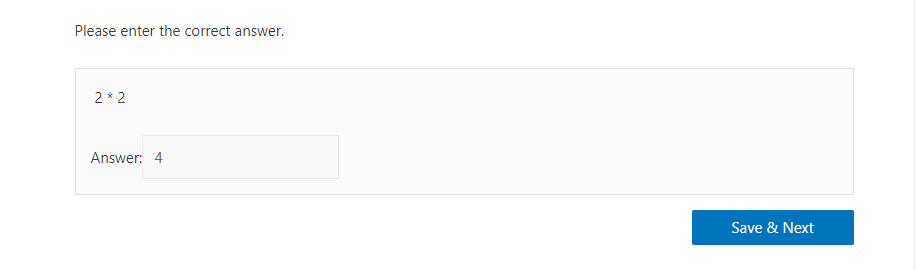Division Questions:

• Navigate to the Questions under the LearnDash menu and click on “Add New”.
• Enter the question title and description.
• Scroll to the Answer(required) section.
• Enter the Question i.e 14 / 2 = _______
• Enter the Formula i.e [x] / [y]
• For this example, enter x as the variable and enter 14 both for the Min and Max values.
• For this example, enter y as the variable and enter 2 both for the Min and Max values.

Note: If you want to randomize the values for a variable, insert the selected value range in min and max fields.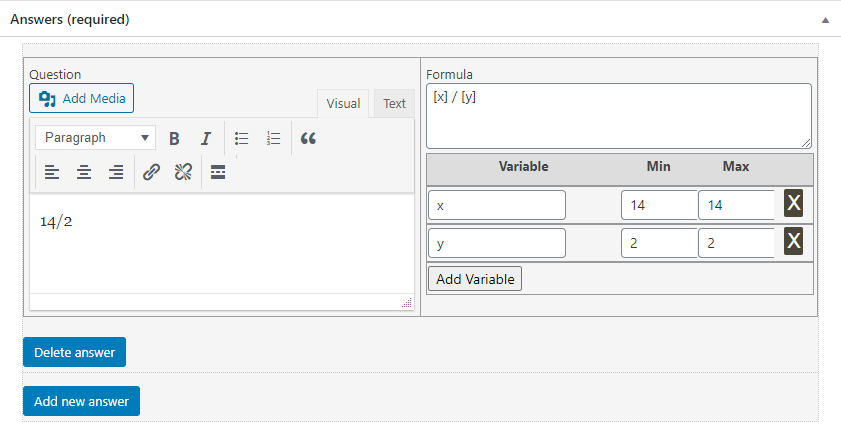This is how the question will be displayed on the front-end: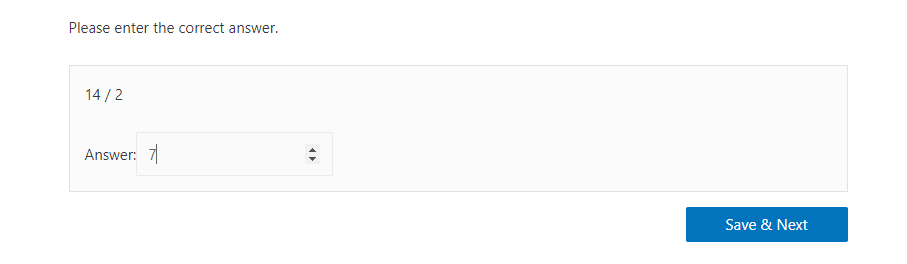Using Maths Functions:

All the functions mentioned below are supported by the Arithmetic question type. Functions should be used in the following format “function(   [a]  ) where “a” is the variable that contains a value.

• cos( [a] )
• sin( [a] )
• tan( [a] )
• abs( [a] )
• exp( [a] )
• log( [a] )
• sqrt( [a] )
• int( [a] )
• round( [a] )
• ceil( [a] )
• floor( [a] )
• fmod( [a] )
• acos( [a] )
• acosh( [a] )
• asin( [a] )
• asinh( [a] )
• atan2( [a] )
• atan( [a] )
• atanh( [a] )
• bindec( [a] )
• cosh( [a] )
• decbin( [a] )
• dechex( [a] )
• decoct( [a] )
• hexdec( [a] )
• log10( [a] )
• max( [a] )
• min( [a] )
• octdec( [a] )
• pi( [a] )
• rand( [a] )
• sinh( [a] )
• rand( [a] )
• srand( [a] )
• tanh( [a] )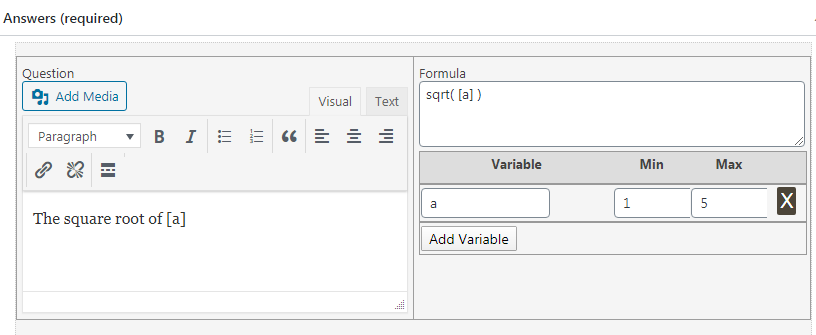Using Decimal Points:

You can use decimal points in “Arithmetic” questions, so that answers will be calculated according to the specified number of decimal points.

You can set the number of decimal points to be used from the meta box that is  shown on the right side of a Arithmetic question’s edit page. If you would like to omit the use of decimals, simply check the “Disable Decimal Values for Answers” box.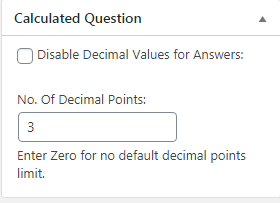This is how a question with answers set to three decimals will be displayed on the front-end: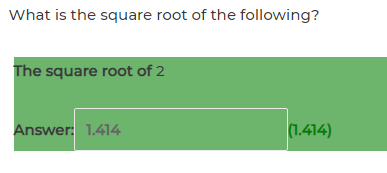Trigonometry Questions:

• Navigate to the Questions under the LearnDash menu and click on “add new”.
• Enter the question title and description.
• Scroll to the Answer(required) section.
• Enter the Question i.e sin( [a] ) = _______
• Enter the Formula i.e sin( [a] )
• For this example, enter a as the variable and  8 for both the Min and Max values. (If you want to randomize the values of the variables used, insert the selected value range in min or max fields).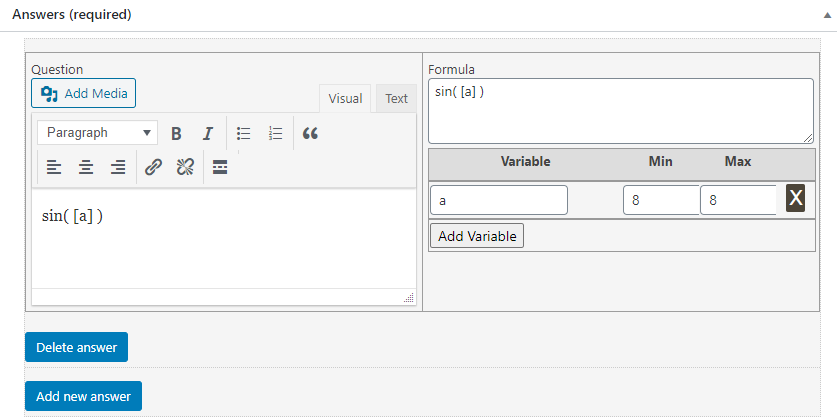This is how the question will appear on the front-end: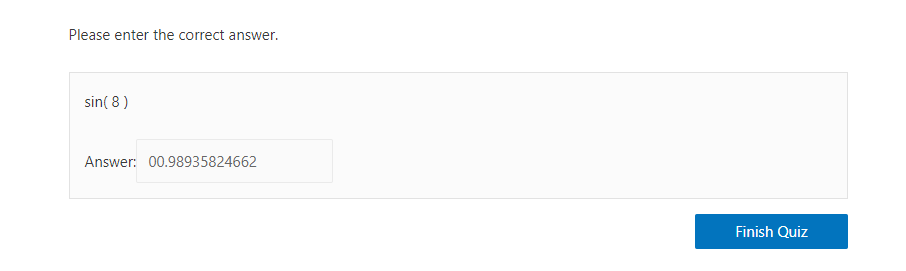Numerical Expression Questions

• Navigate to the Questions under the LearnDash menu and click on “add new”.
• Enter the question title and description.
• Scroll to the Answer(required) section.